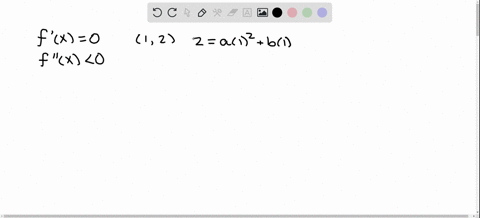Enroll in one of our FREE online STEM bootcamps. Join today and start acing your classes!View Bootcamps### Determine the values of constants $a$ and $b$ so …

02:06Georgia Southern University
Problem 70

# Find the open intervals on which the function $f(x)=a x^{2}+$ $b x+c, a \neq 0,$ is increasing and decreasing. Describe the reasoning behind your answer.

## Discussion

You must be signed in to discuss.

## Video Transcript

all right, so we want to find the open intervals for which the function F of X is a squared plus B X, Let's see, is increasing or decreasing in or assuming that it is not zero. So it's a proper quadratic function. Okay, so Melissa's not so bad. We're just gonna take the derivative and we get two X plus being. And when is F prime equal to zero? What's when X is? This should look familiar. Ah, negative. B over to bang. So that's the location. The vertex of the problem, right? So that's going to either be the local Max or local men, depending on whether a is positive or negative. So we split into two cases. If a is greater than zero, then what we do is we look at sand, so we canoes, they can actually factor out. Well, it's something that so that is greater than zero. Here we have no need to be over too, eh? And if we plug in negative B over two A. If we plug in something larger that need to be over two and a is positive, this is going to be positive and therefore deployments and make smaller, negative B over two days. It's going to be negative because it's going to be less than being pointing negative something smaller than they need to be over, too, eh? And then it is bigger than zero. Sorry, bays lessons. Your exact opposite phenomenon is going to happen when we pulled in something larger than thing to be over, too, eh? Whereas before it was going to be positive. Now it's going to be negative because A is negative. So it's going to switch the sign here and then when your lesson they're going to be over today, where is when he was positive this was going to end up giving you something smaller than being said the turret of the negative. But since a is now negative, that is not switched, okay? And this is what we've come to expect. So if a is greater than zero, we know we have ah, right side up problem soon that matches with decreasing than increasing. And when a lesson zero be an inverted proble. You know I can't disconcert stint with the truth, being positive and the negative. So if we want the said the question asked to find the open intervals for which functions increasing or decreasing. So if a is greater than zero, it's increasing from negative B over two A to infinity and then decreasing from Meghan Infinity to negative B over two A. In today's lessons. Europe. It's increasing frowned negative infinity negative B over today and then decreasing. Who? Meg B over two A. To infinity.